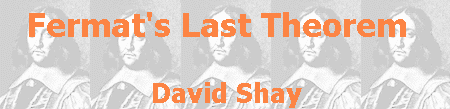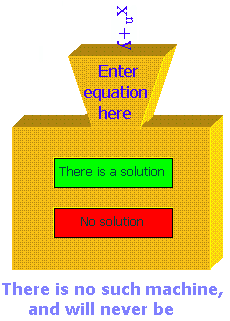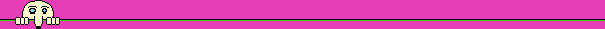## There are bigger problems

With all the fuss about FLT, we should keep in mind that it is dealing with only one Diophantine equation, of which many others exist. Finding general tools to solve Diophantine equations is, of course, much more important than the solution of one specific equation. Kummer, whom no one doubts his contribution to proving FLT, expressed himself radically about this subject, and said that FLT is "more a curiosity than a pinnacle of science". Even though activity to prove FLT directly was continued all along the twentieth century, a new direction emerged: stating new problems, much vaster than FLT, the solution of which will also solve FLT immediately, as a special case.

This approach to solving mathematical problems was presented clearly by Hilbert, in a famous lecture he gave at the international conference of mathematics, which took place in Paris in 1900:

"If we do not succeed in solving a mathematical problem, the reason frequently consists in our failure to recognize the more general standpoint from which the problem before us appears only as a single link in a chain of related problems. After finding this standpoint, not only is this problem frequently more accessible to our investigation, but at the same time we come into possession of a method which is applicable also to related problems. The introduction of complex paths of integration by Cauchy and of the notion of the IDEALS in number theory by Kummer may serve as examples. This way for finding general methods is certainly the most practicable and the most certain; for he who seeks for methods without having a definite problem in mind seeks for the most part in vain."

Faithful to this approach, Hilbert continued his lecture and presented a problem much more general than FLT. In this lecture, probably the most well known lecture in the history of mathematics, Hilbert presented 23 problems, which he believed were central to the development of mathematics. Those problems, whose influence on mathematical research was recognized all over the twentieth century, were identified by proper names. One of them became known by its serial number in Hilbert's list. Hilbert's tenth problem asked to find an algorithm (a process that ends after a finite number of steps), which will enable to determine, about any given Diophantine equation, whether it has a solution. Such an algorithm will be sufficient to prove FLT or disprove it. In case that the algorithm disproves FLT, namely it determines that Fermat's equation has a solution in integers, we may also want to know the values of this solution, but this answer is behind the scope of the algorithm.

 Hilbert gave his lecture in German, but you can read an English translation of it, including all the 23 problems.

Algorithms of the type required by Hilbert, dealing with simple Diophantine equations, were found by Euclid and by Gauss. Therefore, the attempt to find such a general algorithm seemed reasonable. Nevertheless, in 1970 the Russian mathematician Yuri Matiyasevich, aged 22, proved that such an algorithm could not exist.As we learn from our story, mathematical creation evolves not only through step-by-step progress of proving a new theorem from existing ones, but also through far-reaching conjectures, that demand many years of research. Proving a successful conjecture provides a significant breakthrough in mathematical research. Many times, even when the conjecture is disproved, it is still useful because of the new methods used to make it and also because of the meaning of its negation.

In an article published in 1978, Harold Edwards of the University of New York, author of the book Fermat' Last Theorem - A Genetic Introduction to Algebraic Number Theory, wrote: "At the present time, however, the subject of Fermat's last theorem is more or less dormant". That is because any new direction to attack the problem was not found, and the progression in the way formed by Kummer 130 years earlier had ceased. Hundreds of researches broadened the knowledge about FLT, but did not reach a full proof. Absolutely different ways of attacking FLT had started to sprout before Edwards wrote his description, but their potential has been realized years after.

In 1922, a year after the publishing of his book Three Lectures on Fermat's Last Theorem, the British mathematician Louis Mordell made a conjecture that was named, naturally, "Mordell's conjecture". Similarly to Hilbert's tenth problem, Mordell's conjecture does not refer to any specific Diophantine equation, but to Diophantine equations in general. This conjecture is part of the mathematical branch of Algebraic Geometry, and it connects the number of solutions a Diophantine equation has with the shape of the graph of this equation.

In a lecture he gave in 1972, Weil talked with criticism about the chance to prove the conjecture. Here are his words about the conjecture's content: "It would be nice if this were so, and I would rather bet for it than against it. But it is no more than wishful thinking because there is not a shred of evidence for it, and also none against it. In the old days, the word conjecture was reserved (and I suggest that it might still be usefully reserved) for the case when there is some reasonably convincing evidence." However, in 1983 Gerd Faltings, a 29-year-old German mathematician, succeeded to prove Mordell's conjecture (and two other important conjectures). Mordell's conjecture required therefore 69 years to be proved.

 André Weil, Two Lectures on Number Theory, Past and Present, L'Enseignement Mathematique, 1974.

 Should Mordell's conjecture be renamed as Faltings' Theorem? It is not just a question about honor and tradition. This question reflects the place of conjectures in the advancement of mathematics. Nobody will deny that a new theorem emerges from a conjecture, but the dispute focuses on the question how many theories we should build around unproven conjectures. Aren't we creating a house of cards by presenting a conjecture upon conjecture instead of presenting a proof based on other proofs? Nevertheless, when a far-reaching conjecture is finally proved, mathematical knowledge jumps forward. The breakthrough in his proof granted Faltings the Fields medal, "the Nobel prize of mathematics", which is given every four years to the best mathematicians under the age of 40. In 1990 this prize was given to Edward Witten, a physicist from Princeton University, for original conjectures he had made in the mathematical branches of topology and knot theory, conjectures that had triggered major mathematical developments. Some criticizers were arguing that someone who creates proofs, not someone who creates conjectures, should win the prize. Mordell's conjecture remarkably delimits the problem of proving FLT, because it follows from this conjecture that for every power greater than 2 the equation xn+yn=zn has only a finite number of basic solutions, and not a potentially infinite number of solutions, as it was till this conjecture was proved. This finite number may be 0, and thus FLT will be proved. It may be greater than 0, and in this case FLT will be disproved. Nobody has found a way to determine how many solutions there are, so the problem created by Fermat still holds. Moreover, Mordell's conjecture says nothing about the number of powers that have a solution to Fermat's equation; it deals only with the number of solutions for each power separately. Faltings' proof of Mordell's conjecture was described as "opening a new chapter in number theory". In addition to its direct contribution to the proof of FLT, Faltings' work encouraged hope that the modern methods he used will be useful in proving FLT as well.